Home | Study Links | Poems |

## A Cubical Universe, a New Prime Numbers HypothesisWhile searching for prime number patterns, I imagined a line length consisting of an infinite number
of evenly spaced prime numbers (1 to infinity + 1), where infinity = 0, which implies a reversal of
numbers. With other words, the beginning is the end, and the end is the beginning.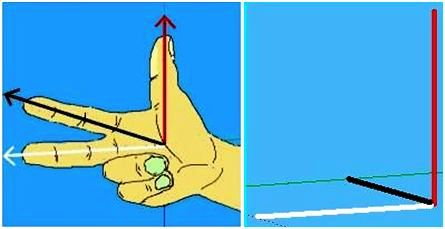Naturally the ends of this line must relate to a mirror image of itself, which brought into play the idea
of Fleming's right-hand rule, which shows the relationship of electromagnetic currents and motion.
The thumb represents motion, the forefinger magnetic field, and the middle finger electric current
at a right angle to the forefinger, so that all are set at right angles to each other.
This is one out of eight identical triune corner pieces.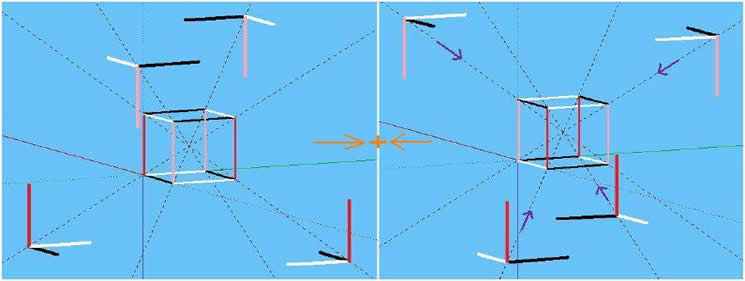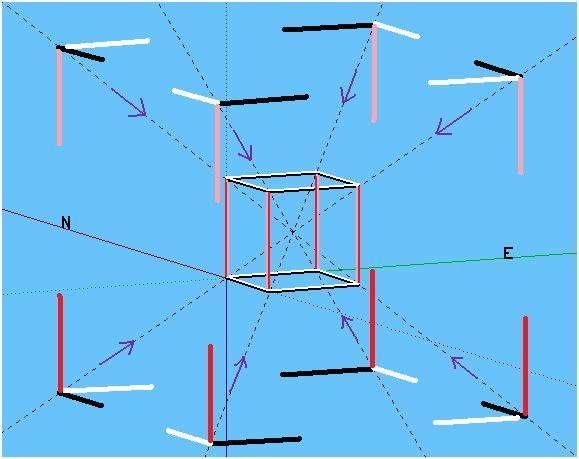Together there are 12 lines making up this cube frame with 12 lines mirroring it.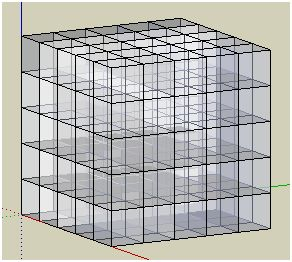Perhaps, the cosmos is comprised of a harmonious grid matrix, interwoven by electromagnetic
spectrum frequency waves comprised of non-prime numbers like finely woven threads of fabric.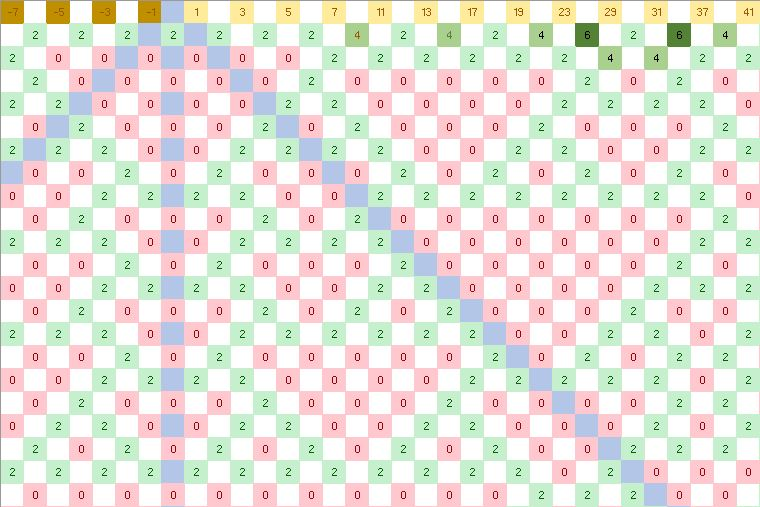The prime numbers reflect a unique and rock-solid three-part pattern, which consists
of (0’s and 2’s), and the white squares that make up half of this pattern. Logically,
the pattern (0’s and 2’s) carrying those prime numbers cannot be out by one iota.First 150 or so prime numbers, and showing the fold.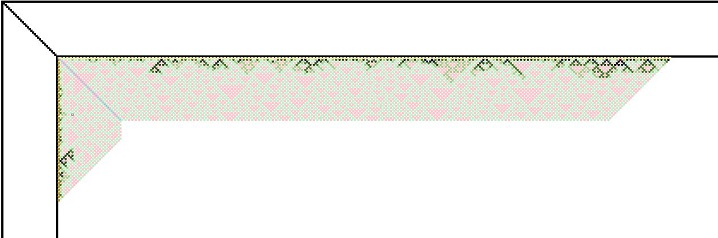The cube frame edges have condensed patterns like waves on the surface of an ocean.
Imagine this as concentrated energy, visible matter, or various sized objects floating on water.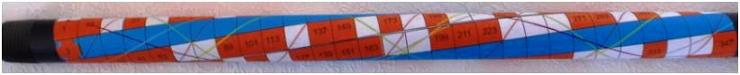The frame edges comprised of prime number frequencies as explained in Primes and Patterns,
are accurately depicted in this image as tubular, circular, and spiralling, instead of flat and straight.The cube represents an infinite universe's' with the center named "big bang". For easier viewing
I omitted the front and back cones. Each of these six cones represents an "expanding" universe.
Each cone has an identical cone that mirrors it. Three pairs, left and right, front and back,
and top and bottom. This is just one cube out of how many?
For more ideas on this multi-dimensional drawing see the Sun Square page.Imagine this cube idea as spherical (atom or universe) where the information originates from the
center. A universe that consists of visible substance as represented by the numbered squares,
with the rest comprised of zeroes representing frequency waves (dark energy), and the empty
squares an invisible grid matrix (dark matter). A tripartite universe that operates with computer like
precision, using digital processes; bits like the 1’s & 0’s of computers, portrayed here as 0’s and 2’s.
Logically an expanding universe already consists of pre-existing ever-growing numbers, frequencies,
patterns, and primes, and giving the appearance of speeding up the further out you look.

Lastly, perhaps the cubical/sphere configuration and the relationship of electromagnetic currents
and motion as described above, could be a pattern for a levitating craft, where an electromagnetic
force is able to manipulate the gravitational force in all directions.
The only force you need to counter is the electromagnetic force Nikola Tesla

Return to top